# 2D Harmonic Oscillator Quantum numbers

## Homework Statement

In the exercise, we solved the 2D Harmonic Oscillator in kartesian (x,y) and polar (r,φ) coordinates.
We found out that both have the same energy levels, but they look very different, when I plot them.
What am I missing? The polar solution seems more like it.

## Homework Equations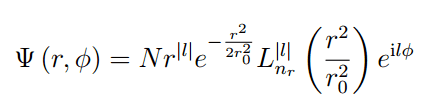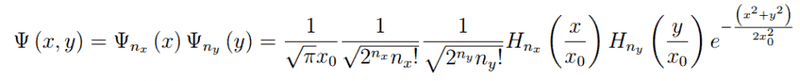## The Attempt at a Solution

Two examples: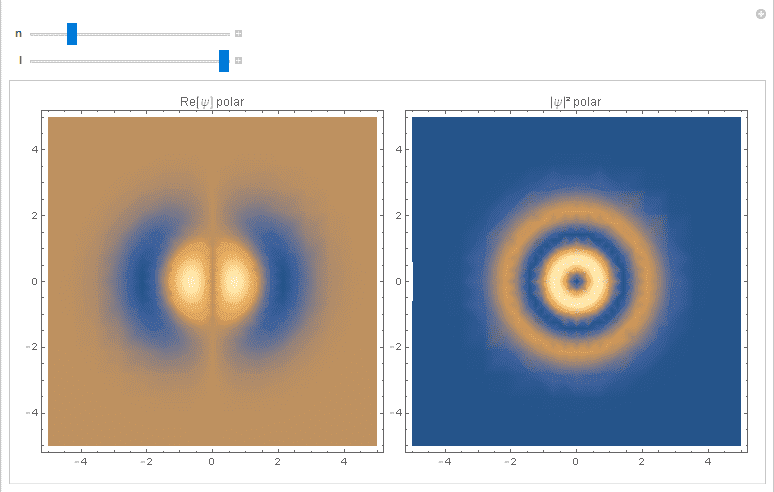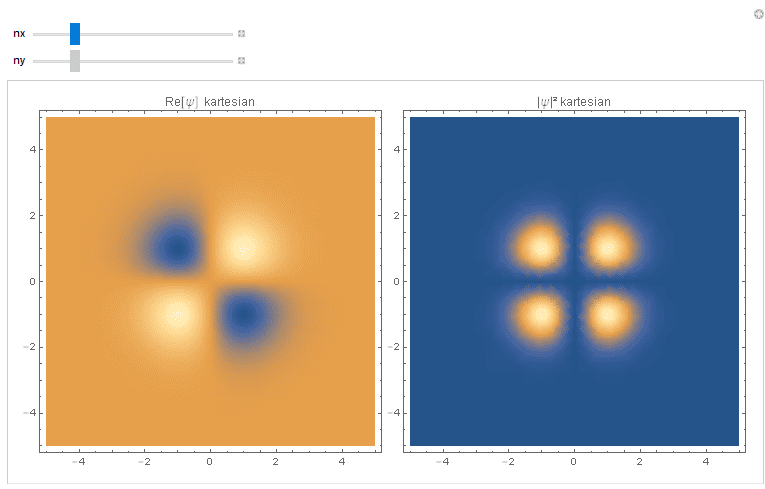If needed, I can give you more details about the formulas or plots!

## Answers and Replies

Homework Helper
What are the corresponding quantum numbers ##(n_x,n_y)## in the case of Cartesian and ##(n,|l|)## in the case of polar of the picture you plot there?

They correspond to the formulas above.
$n_{x}$ and $n_{y}$ are the quantum numbers from the 1D solution.
In the polar case, $n_{r}$ corresponds to the energy and $l$ to the angular momentum.

Last edited:
Homework Helper
They correspond to the formulas above.
nx and ny are the quantum numbers from the 1D solution.
In the polar case, n corresponds to the energy and l to the angular momentum.
What I asked is what values of ##(n_x,n_y)## you used for plottingand what values of ##(n,l)## for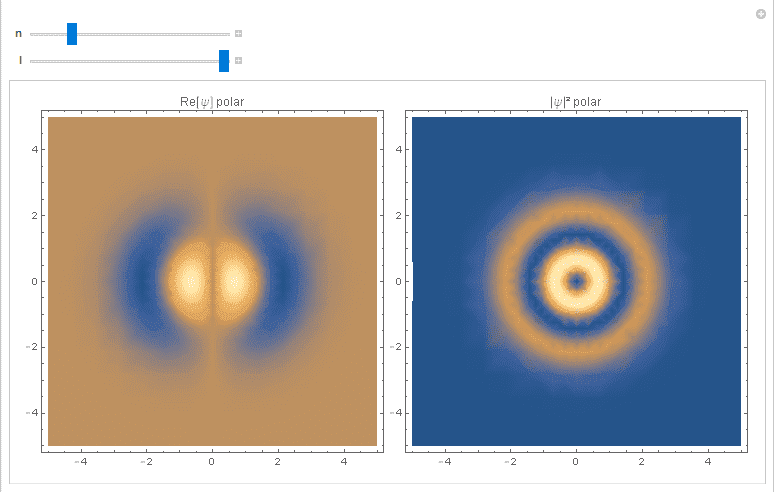?

Oh, sry.. (1,1) in both cases!
They look the same for the ground state.
Should I upload the file?

Homework Helper
Oh, sry.. (1,1) in both cases!
That's the first mistake you made. Polar and Cartesian representations of 2D harmonic oscillator are different sets of bases, the one in polar coordinate is invariant under rotation while the one in Cartesian is not. This means, you should not assume that two pair of quantum numbers in polar and Cartesian representations having identical numerical values to be identically equal to each other. However, they are indeed connected if the energy eigenvalue are the same in both representation, in particular one eigenstate in one representation will be a linear combination of the eigenstates in the other representation. See the table in the last page in this link http://www.physics.rutgers.edu/~steves/501/Lectures_Final/Lec17_Polar Coordinates.pdf.
You can find there too that in polar coordinate the energy eigevalue is given by
$$E_n = \hbar\omega(n+1)$$
while in Cartesian coordinate by
$$E_{n_x,n_y} = \hbar\omega(n_x+n_y+1)$$
Here you can see that for the same energy in polar and Cartesian coordinates, the quantum numbers are related by ##n=n_x+n_y##. Using your program, try plotting the real part of ##(n,l) = (1,1)## for polar and ##(n_x,n_y) = (1,0)## in Cartesian.

•Actually, it is $n=n_{x}+n_{y}=2n_{r}+l$.
But I tried many different combinations, they behave very different in general.
(The polar solution has circular symmetry and the cartesian has mirroring symmetry)

Homework Helper
But I tried many different combinations, they behave very different in general.
Indeed, they should be different. You can't find any pair of eigenfunctions in polar and in Cartesian which look identical as an eigenstate in one coordinate is a linear combination of eigenstates in the other coordinates, as I have mentioned in my previous comment. The only identical pair is the ground state because this energy level has no degeneracy. In fact, the eigenstate in polar is complex on the other hand the one in Cartesian is always real, no way to compare them one-on-one.
(The polar solution has circular symmetry and the cartesian has mirroring symmetry)
I have also pointed out this point in my previous comment. The polar representation is also eigenstate of angular momentum operator, which means it must indeed have circular symmetry. The Cartesian representation is eigenstate of ##x## and ##y## parity operators, so they will have mirror symmetry as you already observed.

•